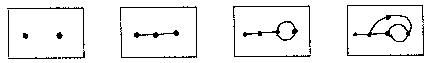Back to Graph Theory

## Rules

Start with a few dots (vertices). For the first few games, start with 2-4 vertices. Players take turns connecting two vertices with a curve (edge) and placing a new vertex along this edge. This is done following two rules:

1. Each vertex can have at most three edges coming from it
2. Edges must be drawn so that they do not cross or touch any other vertices than the two they are connecting

If a player is not able to draw an edge according to the rules, the other player wins. NOTE: you can draw an edge connecting a vertex to itself, such as:A game might start like:Play a few games and then check out the questions below!

## Questions

(not all questions have final answers!)

1. What can the ending look like? The degree of a vertex is how many edges go to the vertex. Can you play a game so that each vertex ends up with degree 3? Can you play a game that ends with two degree 2 vertices? Three degree 2 vertices? A degree 1 vertex? Can you describe all the possible sets of degrees a game can end with?